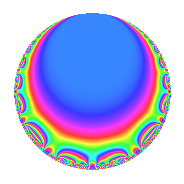# Properties

 Label 33.2.dLevel 33 Weight 2 Character orbit d Rep. character $$\chi_{33}(32,\cdot)$$ Character field $$\Q$$ Dimension 2 Newforms 1 Sturm bound 8 Trace bound 0

# Related objects

## Defining parameters

 Level: $$N$$ = $$33 = 3 \cdot 11$$ Weight: $$k$$ = $$2$$ Character orbit: $$[\chi]$$ = 33.d (of order $$2$$ and degree $$1$$) Character conductor: $$\operatorname{cond}(\chi)$$ = $$33$$ Character field: $$\Q$$ Newforms: $$1$$ Sturm bound: $$8$$ Trace bound: $$0$$

## Dimensions

The following table gives the dimensions of various subspaces of $$M_{2}(33, [\chi])$$.

Total New Old
Modular forms 6 6 0
Cusp forms 2 2 0
Eisenstein series 4 4 0

## Trace form

 $$2q + q^{3} - 4q^{4} - 5q^{9} + O(q^{10})$$ $$2q + q^{3} - 4q^{4} - 5q^{9} - 2q^{12} + 11q^{15} + 8q^{16} - 12q^{25} - 8q^{27} + 10q^{31} - 11q^{33} + 10q^{36} - 14q^{37} + 11q^{45} + 4q^{48} + 14q^{49} + 22q^{55} - 22q^{60} - 16q^{64} - 26q^{67} + 11q^{69} - 6q^{75} + 7q^{81} + 5q^{93} + 34q^{97} - 11q^{99} + O(q^{100})$$

## Decomposition of $$S_{2}^{\mathrm{new}}(33, [\chi])$$ into irreducible Hecke orbits

Label Dim. $$A$$ Field CM Traces $q$-expansion
$$a_2$$ $$a_3$$ $$a_5$$ $$a_7$$
33.2.d.a $$2$$ $$0.264$$ $$\Q(\sqrt{-11})$$ $$\Q(\sqrt{-11})$$ $$0$$ $$1$$ $$0$$ $$0$$ $$q+\beta q^{3}-2q^{4}+(1-2\beta )q^{5}+(-3+\beta )q^{9}+\cdots$$